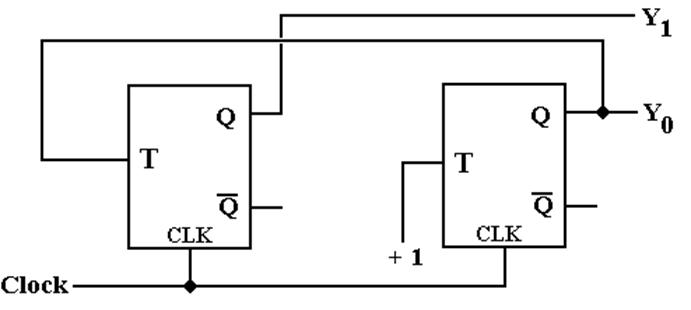Combinational vs. Sequential Circuits.

Basically, sequential circuits have memory and combinational circuits do not.

Here is a basic depiction of a sequential circuit.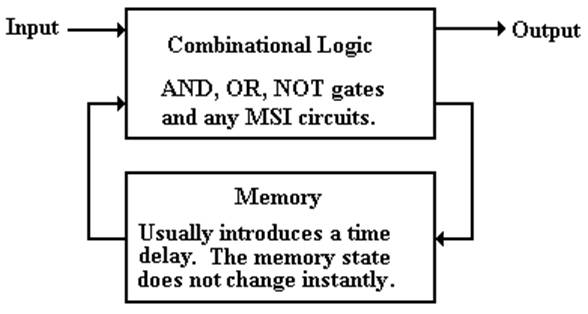All sequential circuits contain combinational logic in addition to the memory elements.

We now consider the analysis and design of sequential circuits.

Finite State Machines: Notation

In this course, we represent sequential circuits as finite state machines.

A Finite State Machine (FSM) is a circuit that can exist in a finite number of states, usually a rather small number.  Finite State Machines with more than 32 states are rare.

The FSM has a memory that stores its state.

If the FSM has N states, then its memory can be implemented with P flip–flops where

2P–1 < N £ 2P

Typical values:               3 states    2 flip–flops

4 states    2 flip–flops

5 states    3 flip–flops

8 states    3 flip–flops

Tools to describe finite states machines include

1)     The state diagram

2)     The state table

3)     The transition table

State Diagram for a Sequence Detector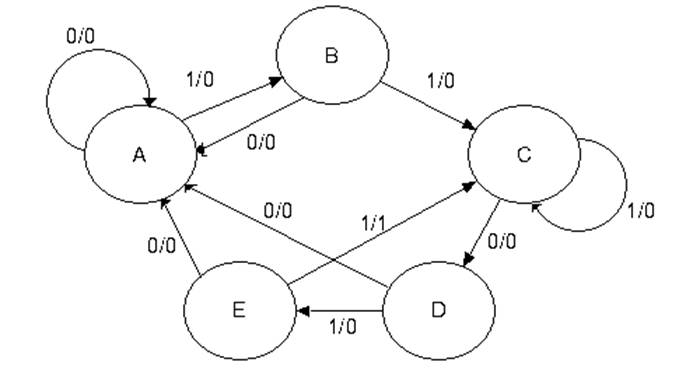NOTE:    We have five states, labeled “A”, “B”, “C”, “D”, and “E”.

We have labeled edges connecting the states.  Each is labeled Input / Output.

This is a directed graph with labeled edges and loops.

More Notes on the State Diagram

1.     The main function of the state diagram for the FSM is to indicate what the
next state will be given the present state and input.

2.     Here the input is labeled X.  Were the input two bits at a time,
the input would be labeled as X1 X0, with X1 the more significant bit.

3.     The labeling of the arcs between the states indicates that there is output
associated with each transition.  Not all Finite State Machines have
output associated with the transition.  This one does.

4.     This and all typical FSM represents a synchronous machine.
Transitions between states and production of output (if any) takes place at a fixed
phase of the clock, depending on the flip–flops used to implement the circuit.

5.     Were we pressed to be more specific, we would associate the transitions
with the rising edge of the clock.  This is usually an unnecessary detail.

State Diagram for a Modulo–4 Counter

Here is the state diagram for a modulo–4 counter.

There is no input but the clock.  It just counts clock pulses.

Note the direction of the arrows; this is an up–counter.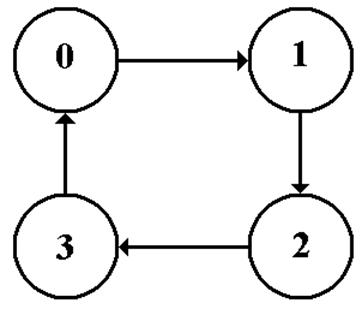State Tables

The state table is a tabular form of the state diagram.  It is easier to work with.

Here is the state table for the sequence detector.

 Present State Next State / Output X = 0 X = 1 A A / 0 B / 0 B A / 0 C / 0 C D / 0 C / 0 D A / 0 E / 0 E A / 0 C / 1

Here is the state table for the modulo–4 counter.

 Present State Next State 0 1 1 2 2 3 3 0

Transition Tables

Transition tables are just state tables in which the labels have been replaced by binary numbers.  Often the labels are retained to facilitate translation to binary.

Here is the transition table for the sequence detector.

 Present State Next State / Output X = 0 X = 1 A = 000 000 / 0 001 / 0 B = 001 000 / 0 010 / 0 C = 010 011 / 0 010 / 0 D = 011 000 / 0 100 / 0 E = 100 000 / 0 010 / 1

Here is the transition table for the modulo–4 counter.  There is no output table.

 Present State Next State 0 = 0 0 0 1 1 = 0 1 1 0 2 = 1 0 1 1 3 = 1 1 0 0

Sample Circuit for Analysis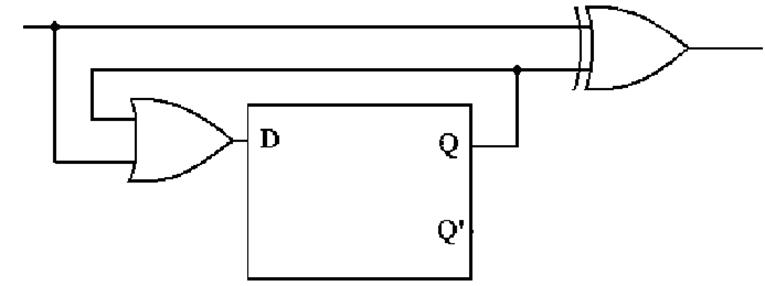The analysis of such a circuit follows a fixed set of steps.

1)     Determine the inputs and outputs of the circuit.  Assign variables to represent these.

2)     Characterize the inputs and outputs of the flip-flops.  Show as Boolean expressions.

3)     Construct the Next State and Output Tables.

4)     Construct the State Diagram.

5)     If possible, identify the circuit.  There are no good rules for this step.

Step 1: Determine the inputs and outputs of the circuit.

The circuit has one input and one output, with one internal variable of interest.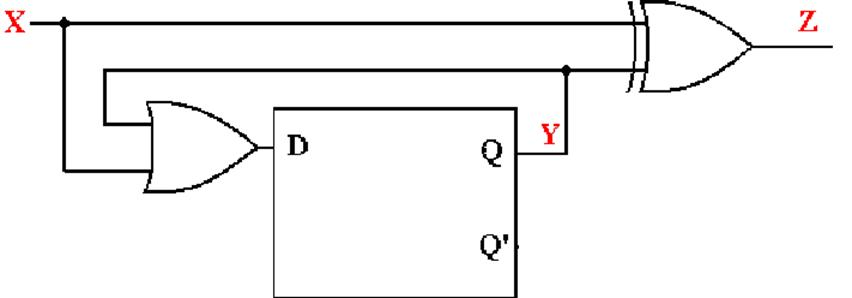The input is labeled as X.

The output is labeled as Z.

The internal line that is fed back into the flip–flop is labeled as Y.

NOTE:    There is output associated with the input because we see the gate producing
Z based on the input X.

Step 2: Show the inputs and outputs as Boolean expressions.Input:                              X

Output:                            Z = X Å Y

Input to Flip–Flop:         D = X +Y

Output of Flip–Flop:       Y

Step 3: Construct the Next State and Output Tables

Here is the next state table.

 X Q(t) = Y D = X + Y Q(t+1) 0 0 0 0 0 1 1 1 1 0 1 1 1 1 1 1

We know the present state of the flip–flop; call it Y.

Given Y and X, the input, we can compute D.  This determines the next state.

Here is the output table.  It depends on the input and present state.

 X Y = Q(t) Z 0 0 0 0 1 1 1 0 1 1 1 0

Step 3A: Construct the Next State / Output Table

Just combine the two tables into one table.

 X Q(t) = Y D = X + Y Q(t+1) / Z 0 0 0 0 / 0 0 1 1 1 / 1 1 0 1 1 / 1 1 1 1 1 / 0

We then put the table into a standard form that will lead to the state diagram.

 Present State Next State/Output X = 0 X = 1 0 0 / 0 1 / 1 1 1 / 1 1 / 0

We use this to build a state diagram.  The two states are Q = 0 and Q = 1.
The outputs are associated with the transitions.

Step 4: Construct the State Diagram.

Here again is the state table with output.

 Present State Next State/Output X = 0 X = 1 0 0 / 0 1 / 1 1 1 / 1 1 / 0

Here is the state diagram.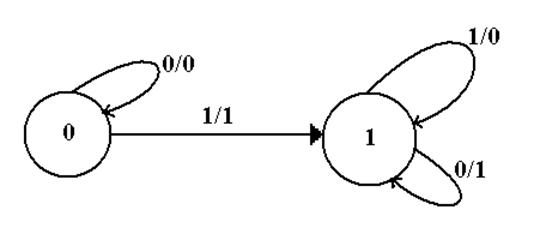Step 5: Identify the Circuit if Possible

This is often hard to do.

The key here is that the circuit stays in state 0 until the first 1 is input.
When the first 1 is input it goes to state 1 and stays there for all input.

We now characterize the output as a function of the input for each of the two states.

Input   Q(T)   Output

0          0           0           For Q(t) = 0, the output is X

1          0           1

0          1           1           For Q(t) = 1, the output is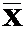.

1          1           0

It can be shown that this is a serial generator for a two’s–complement.

The binary integer is read from Least Significant Bit to Most Significant Bit.

Up to and including the first (least significant) 1, the input is copied.

After that it is complemented.

0001 1100       becomes 1110 0100
0010 1101       becomes 1101 0011.               Try this, it works.

Design of a Sequential Circuit

We begin with the rules for a simple procedure to do the design.

1)     Derive the state diagram and state table for the circuit.

2)     Count the number of states in the state diagram (call it N) and calculate the
number of flip-flops needed (call it P) by solving the equation
2P–1 < N
£ 2P.  This is best solved by guessing the value of P.

3)     Assign a unique P-bit binary number (state vector) to each state.
Often, the first state = 0, the next state = 1, etc.

4)     Derive the state transition table and the output table.

5)     Separate the state transition table into P tables, one for each flip-flop.
WARNING: Things can get messy here; neatness counts.

6)     Decide on the types of flip-flops to use.  When in doubt, use all JK’s.

7)     Derive the input table for each flip-flop using the excitation tables for the type.

8)     Derive the input equations for each flip-flop based as functions of the input
and current state of all flip-flops.

9)     Summarize the equations by writing them in one place.

10)   Draw the circuit diagram.  Most homework assignments will not go this far,
as the circuit diagrams are hard to draw neatly.

Design a Modulo–4 Counter

Step 1: Derive the state diagram and state table for the circuit.

Here is the state diagram.  Note that it is quite simple and involves no input.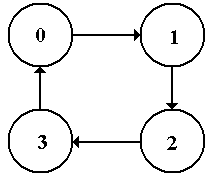Here is the state table for the modulo–4 counter

 Present State Next State 0 1 1 2 2 3 3 0

Step 2: Count the Number of States

Obviously, there are only four states, numbered 0 through 3.

Determine the number of flip–flops needed.

Solve 2P–1 < N £ 2P.  If N = 4, we have P = 2 and 21 < 4 £ 22.

We need two flip–flops for this design.  Number them 1 and 0.

Their states will be Q1 and Q0 or Y1 and Y0, depending on the context.

Remember: 21 = 2, 22 = 4, 23 = 8, 24 = 16, 25 = 32, 26 = 64, 27 = 128, etc.

Step 3 Assign a unique P-bit binary number (state vector)
to each state.

Here P = 2, so we assign a unique 2–bit number to each state.

For a number of reasons the first state, state 0, must be assigned Y1 = 0 and Y0 = 0.

For a counter, there is only one assignment that is not complete nonsense.

 State 2-bit Vector 0 0 0 1 0 1 2 1 0 3 1 1

The 2–bit vectors are just the unsigned binary equivalent of the decimal state numbers.

Step 4 Derive the state transition table.

 Present State Next State 0 00 01 1 01 10 2 10 11 3 11 00

Strictly speaking, we should have dropped the decimal labels in this step.

However, this representation is often useful for giving the binary numbers.

The state transition table tells us what the required next state will be
for each present state.

Step 5 Separate the state transition table into P tables,
one for each flip-flop.

Here P = 2, so we need two tables.

 Flip-Flop 1 Flip-Flop 0 Present State Next State Present State Next State Y1 Y0 Y1( t+1 ) Y1 Y0 Y0( t+1 ) 0    0 0 0    0 1 0    1 1 0    1 0 1    0 1 1    0 1 1    1 0 1    1 0

Each flip–flop is represented with the complete present state and its own next state.

Step 6 Decide on the types of flip-flops to use.
When in doubt, use all JK’s.

Our design will use JK flip–flops.

For design work, it is important that we remember the excitation table.

Here it is.

 Q( t ) Q( t+1 ) J K 0 0 0 d 0 1 1 d 1 0 d 1 1 1 d 0

Step 7 Derive the input table for each flip-flop using the excitation tables for the type.

Here is the table for flip–flop 1.

 PS NS Input Y1 Y0 Y1 J1 K1 0 0 0 0 d 0 1 1 1 d 1 0 1 d 0 1 1 0 d 1

Here is the table for flip–flop 0.

 PS NS Input Y1 Y0 Y0 J0 K0 0 0 1 1 d 0 1 0 d 1 1 0 1 1 d 1 1 0 d 1

Step 8 Derive the input equations for each flip-flop

I use a set of intuitive rules based on observation and not on formal methods.

1)     If a column does not have a 0 in it, match it to the constant value 1.

If a column does not have a 1 in it, match it to the constant value 0.

2)     If the column has both 0’s and 1’s in it, try to match it to a single variable,
which must be part of the present state.  Only the 0’s and 1’s in a column
must match the suggested function.

3)     If every 0 and 1 in the column is a mismatch, match to the complement
of a function or a variable in the present state.

4)     If all the above fails, try for simple combinations of the present state.

NOTE:    The use of the complement of a state in step 3 is due to the fact that
each flip–flop outputs both its state and the complement of its state.

Step 8 Derive the input equations for each flip-flop

Here is the input table for Flip–Flop 1

 PS NS Input Y1 Y0 Y1 J1 K1 0 0 0 0 d 0 1 1 1 d 1 0 1 d 0 1 1 0 d 1

J1 = Y0      K1 = Y0

Here is the input table for Flip–Flop 0

 PS NS Input Y1 Y0 Y0 J0 K0 0 0 1 1 d 0 1 0 d 1 1 0 1 1 d 1 1 0 d 1

J0 = 1        K0 = 1

Step 9 Summarize the equations by writing them in one place.

Here they are.

J1 = Y0             K1 = Y0

J0 = 1               K0 = 1

For homework and tests, this is required so that I can easily find the answers.

Step 10   Draw the circuit diagram.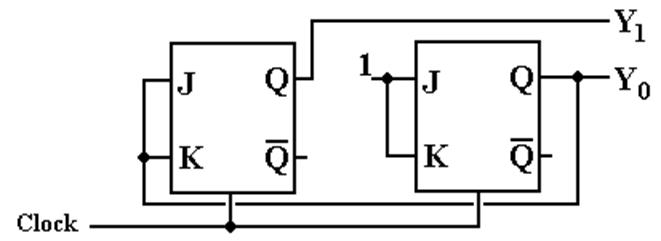But note that each flip–flop has input J = K.  This suggests a simplification.

Step 10A   Draw the circuit diagram again.

Here is a simpler version.